# Zhukovskii function

(diff) ← Older revision | Latest revision (diff) | Newer revision → (diff)

The rational function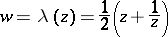of the complex variable. It is important for its applications in fluid mechanics, which were discovered by N.E. Zhukovskii (see , ), particularly in constructing and studying the Zhukovskii profile (Zhukovskii wing). Suppose that a circleis given in the-plane passing through the points(Fig. a), together with a circle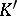touchingon the outside at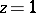, with centreand radius. Under the mapping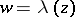, the image ofis a closed curve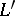with a cusp at the point, touching an arc of the circle(the image of) at that point; this image is represented in Fig. band is the Zhukovskii profile.Figure: z099280a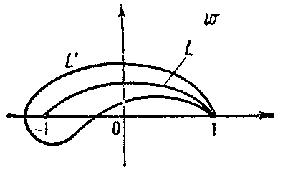Figure: z099280b

The function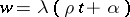maps the exterior of the unit circle in the-plane to the exterior of. To obtain a Zhukovskii profile of a more general shape and disposition, the generalized Zhukovskii function is applied (see , , ):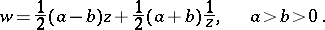How to Cite This Entry:
Zhukovskii function. Encyclopedia of Mathematics. URL: http://encyclopediaofmath.org/index.php?title=Zhukovskii_function&oldid=16495
This article was adapted from an original article by E.D. Solomentsev (originator), which appeared in Encyclopedia of Mathematics - ISBN 1402006098. See original article